# Rounding to the Nearest Hundred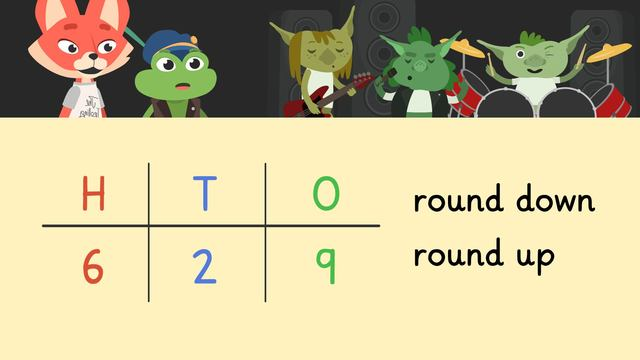Rate this video

Be the first to rate this video!

The authorTeam Digital

## DescriptionRounding to the Nearest Hundred

### Rounding to the Nearest Hundred

Skylar and Henry want to attend a Trolling Stones concert, but tickets are sold out. On their way home, they come across the Tricky Troll on a bridge. He promises Skylar and Henry two spare tickets to the concert if they can solve the rounding to hundred problem he has for them. To solve the problem, they are asked to round a number to the nearest hundred. How do you round to the nearest hundred? Below you will find examples on rounding a number to the nearest hundred.

### How to Round to the Nearest Hundred

When rounding off to the nearest hundred, a place value chart can help you. You will need a place value chart with hundreds, tens, and ones, like the one below.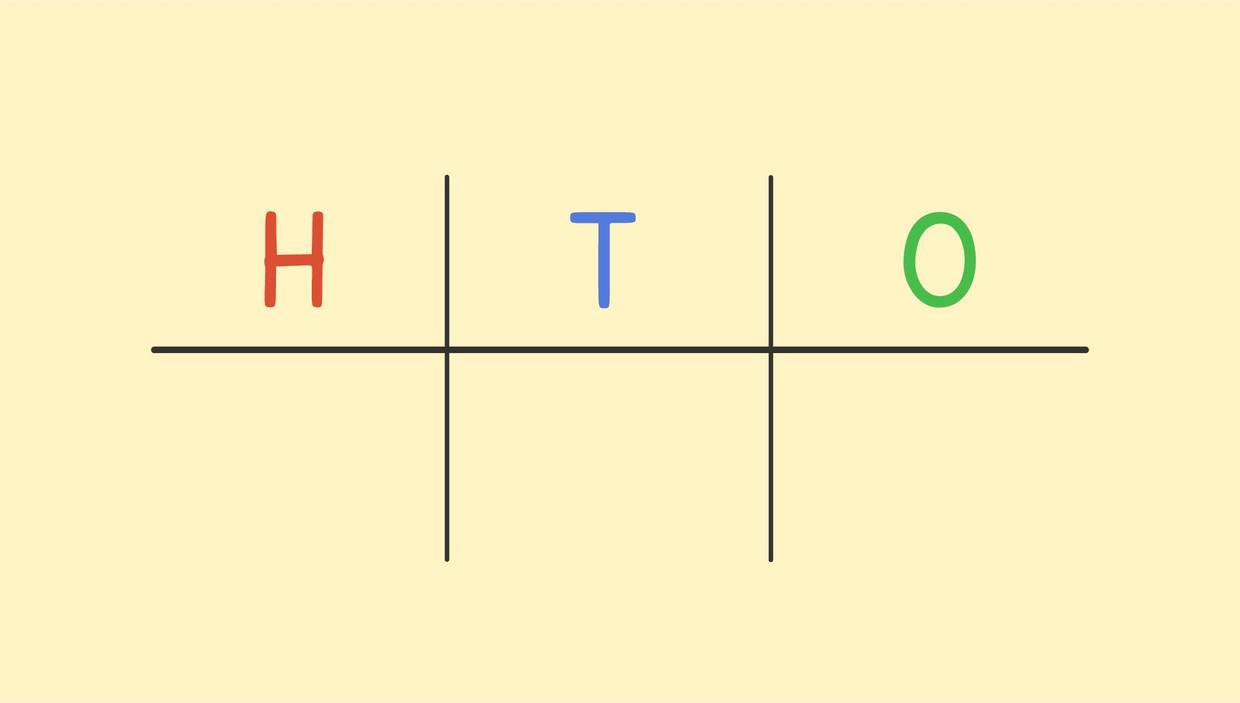#### How do I Round to the Nearest Hundred?

How do you round off to the nearest hundred examples with steps.

First you identify the hundreds place, then look at the place value to the right, which is the tens place. If the number in the tens place is below five, we round down to the nearest hundred and keep the hundreds place the same. If the number in the tens place is five or above, we round up to the nearest hundred, meaning we add one to the number in the hundreds place. Every place value to the right of the hundreds place becomes zero.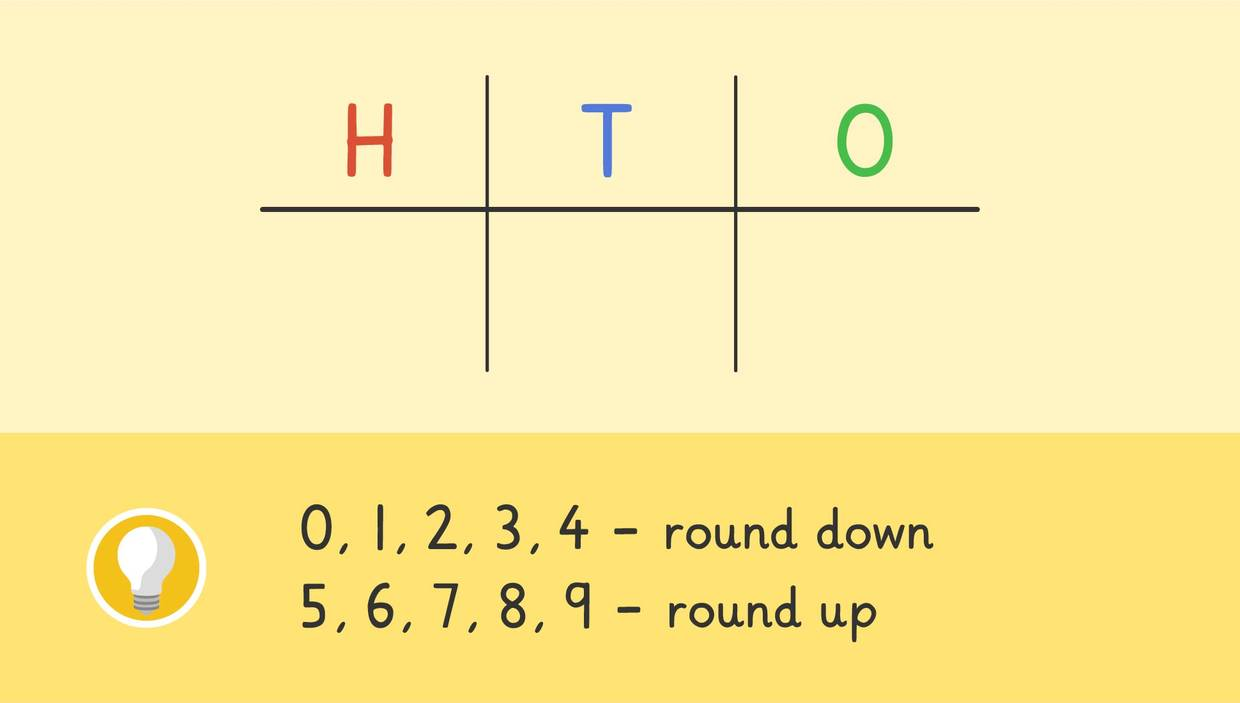### Rounding to the Nearest Hundred Examples

Below you can find some examples of rounding whole numbers to the nearest hundred. One example is a rounding down to the nearest hundred problem, and the other is a rounding up to the nearest hundred problem.

#### Rounding Down to the Nearest Hundred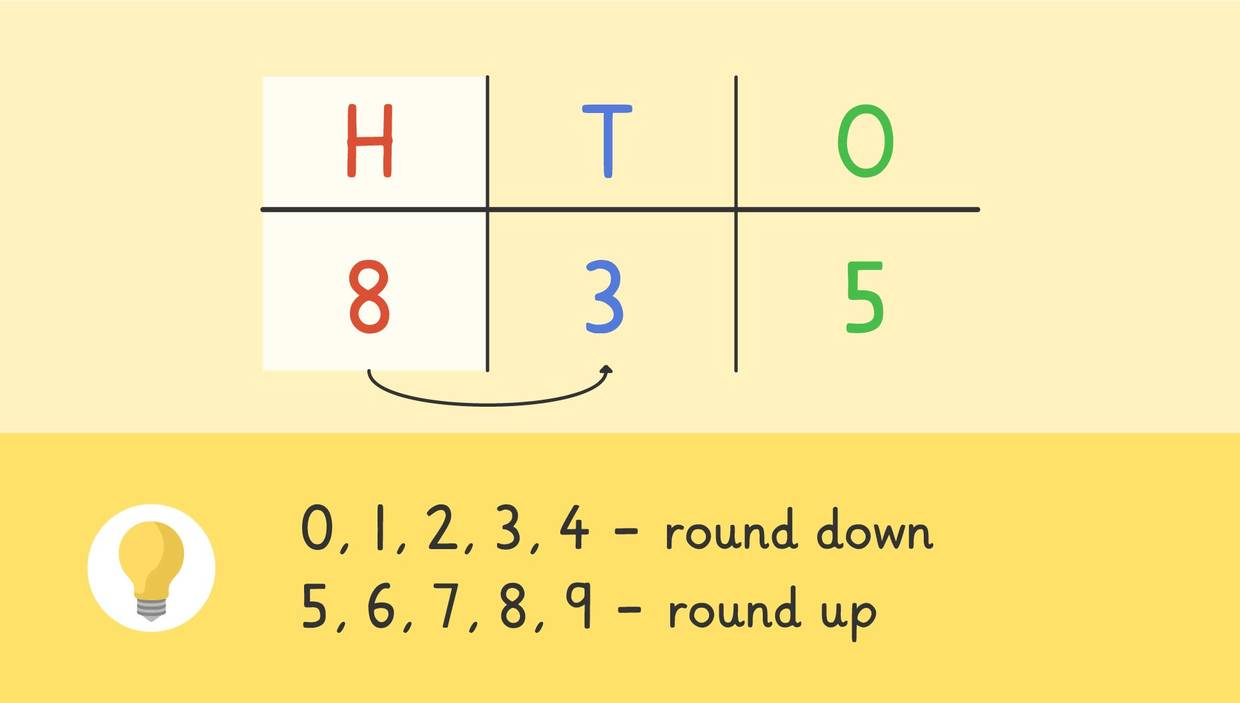The number here is 835. First we identify the number in the hundreds place, which is an 8. Then we go to the place value to the right, the tens place. Here we see a 3. 3 is a number that is below five, so we know we need to round down to the nearest hundred. This means the 8 in the hundreds place stays the same, and we replace the numbers in the tens and ones place values with zeroes. 835 rounded to the nearest hundred is 800.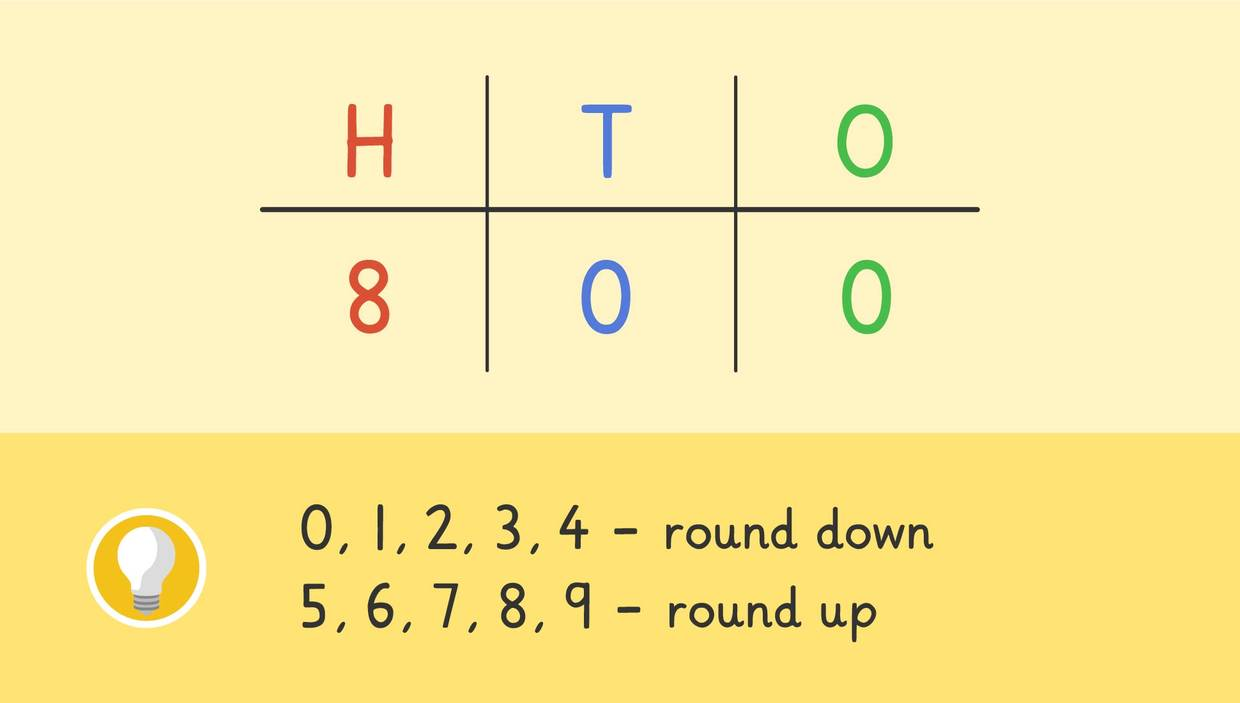#### Rounding Up to the Nearest Hundred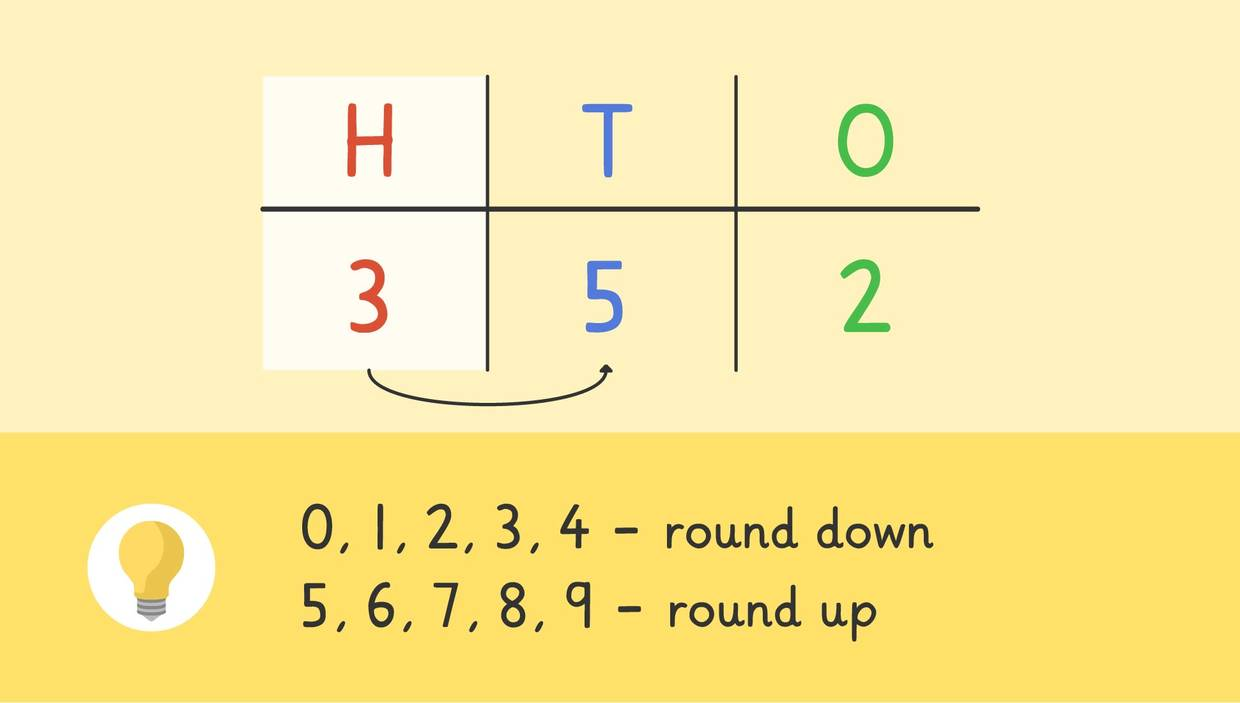The number here is 352. First we identify the number in the hundreds place, which is a 3. Then we go to the place value to the right, the tens place. Here we see a 5. 5 is a number that is five or above, so we know we need to round up to the nearest hundred. This means the 3 in the hundreds place becomes a 4 because we add one to it, and we replace the numbers in the tens and ones place value with zeroes. 352 rounded to the nearest hundred is 400.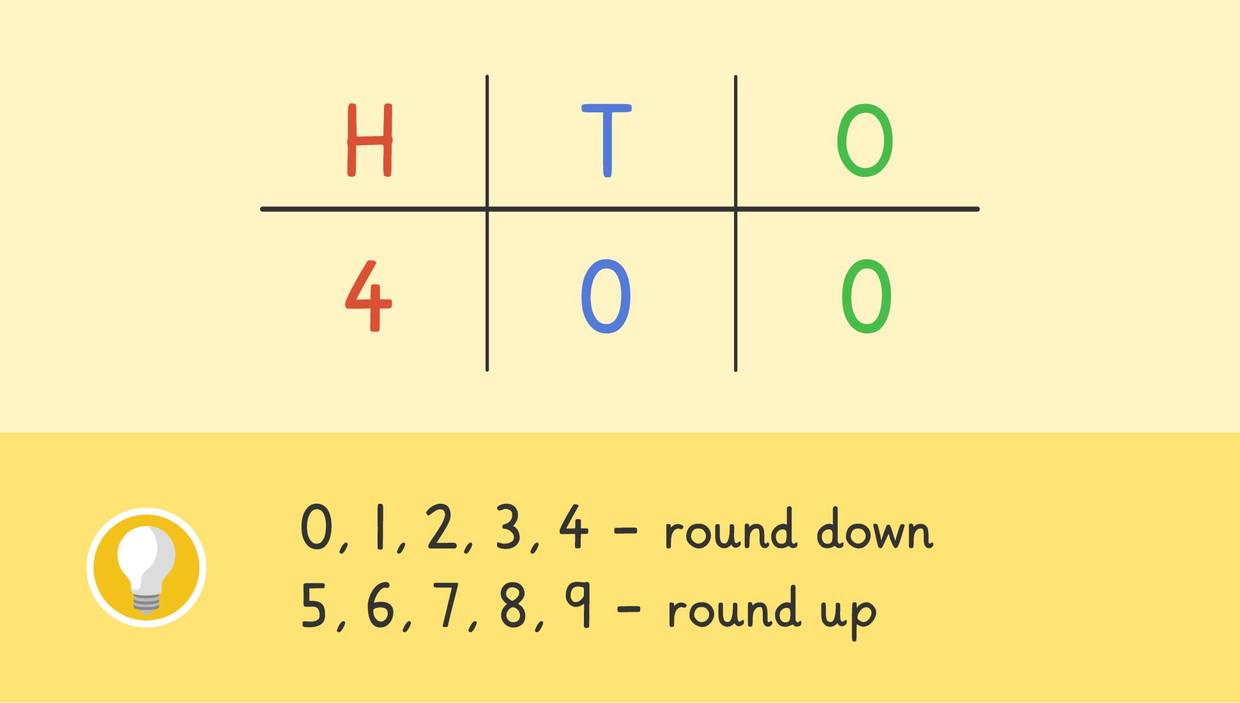### Rounding to the Nearest 100 Summary

To round to the nearest 100, you can set up a place value chart to help you. Then follow these steps to round to the nearest hundred:

• Identify the hundreds place number
• Look at the number to the right in the tens place value
• If the tens place number is below five, round down
• If the tens place number is five or above, round up *If you are rounding down, keep the number in the hundreds place the same and replace the tens and ones place with a zero
• If you are rounding up, add one to the number in the hundreds place and replace the tens and ones place with a zero

You can also find rounding to the nearest hundred worksheets below.

## #

### TranscriptRounding to the Nearest Hundred

Skylar and Henry want to attend a concert, but all the tickets are sold out! Going home, they come to the bridge they need to cross. Wait, who is that standing on the bridge? It's the Tricky Troll! "I have a rounding to the nearest hundred problem for you both...and if you solve it, I shall give you two tickets to the concert!" "Rounding to the nearest hundred" When rounding to the nearest hundred, we can use a place value chart like this to help us set up our Hundreds, Tens, and Ones columns. And then we look at the place value to the right, which is the tens place. If the number in the tens place is below five, we round down to the nearest hundred and keep the hundreds place number the same, but if the number in the tens place is five or above, we round up to the nearest hundred, meaning we add one to the number in the hundreds place. Every place value to the right of the hundreds place becomes zero. Let’s look at some examples. Here we see the number eight hundred and thirty-five. First let's identify the hundreds place. Do you know which number is in the hundreds place? Eight is in the hundreds place. Next go to the place value to the RIGHT of the hundreds place which is the tens place. What number is in the tens place? Three is in the tens place. Three is a number below five, so we round down to the nearest hundred. This means the hundreds place stays an eight and we replace both the tens and ones place with zeros. Eight hundred and thirty-five rounded to the nearest hundred is eight hundred. It is closer to eight hundred than it is to nine hundred! Here is another example. The number is three hundred and fifty-two. First, let's identify the hundreds place. What number is in the hundreds place? Three is in the hundreds place. Now we look at the place value to the right of the hundreds place. What number is in the tens place? Five is in the tens place. Five is a number "five or above", meaning we round up to the nearest hundred. This means the hundreds place becomes a four because we add one to the three and we replace the tens and ones places with zeros. Three hundred and fifty-two rounded to the nearest hundred is four hundred. It is closer to four hundred than it is to three hundred! Now that you know how to round numbers to the nearest hundred using place value. Let's see if you can help Skylar and Henry win concert tickets from the Tricky Troll. The Tricky Troll gives them a number to round, which is six hundred and twenty-nine. What is the first step you need to do? First, you identify the hundreds place. What is the number in the hundreds place? Six is in the hundreds place. What is the next step? Next, you look at the number in the tens place. What is the number in the tens place? Two is in the tens place. You know that two is a number below five, so should we round down, or round up? You round down to the nearest hundred. What is six hundred and twenty-nine rounded to the nearest hundred? It’s six hundred! Six hundred and twenty-nine rounded to the nearest hundred is six hundred. It is closer to six hundred than it is to seven hundred. Now the Tricky Troll's problem has been solved, Skylar and Henry can attend the concert! Remember, when rounding to the nearest hundred with place value. We identify the number in the hundreds place. Then look at the place value to the right which is the tens place. If the tens place number is below five, we round down to the nearest hundred. If the tens place number is five or above, we round up to the nearest hundred. Once inside the concert, they are shocked to see that the Tricky Troll is one of the band members!

## Rounding to the Nearest Hundred Exercise

Would you like to practice what you’ve just learned? Practice problems for this video Rounding to the Nearest Hundred help you practice and recap your knowledge.
• ### Round the number of listeners to the nearest hundred.

Hints

To round to the nearest hundred, look at the digit in the tens place.

• If the tens place is 0, 1, 2, 3, or 4, round down.
• If the tens place is 5, 6, 7, 8, or 9, round up.
• When rounding down, the number in the hundreds place stays the same.
• When rounding up, the number in the hundreds place is one more.

For example, the number 832 rounds to 800 because there is a 3 in the tens place.

The number 862 rounds to 900 because there is a 6 in the tens place.

Solution

First, look at the number in the tens place. In 143, the number 4 is in the tens place.

So, 143 will round down. This means the number "1" stays in the hundreds place and will be followed by two 0s.

The number 143 rounds down to 100.

• ### Round the numbers to the nearest hundred.

Hints

To round to the nearest hundred, look at the digit in the tens place.

• If the tens place is 0, 1, 2, 3, or 4, round down.
• If the tens place is 5, 6, 7, 8, or 9, round up.
• When rounding down, the number in the hundreds place stays the same.
• When rounding up, the number in the hundreds place is one more.

For example, the number 832 rounds to 800 because there is a 3 in the tens place.

The number 862 rounds to 900 because there is a 6 in the tens place.

Solution

For each number, first find the tens place. Then, round up or down.

• In the number 701, the number 0 is in the tens place. So, this rounds down and the hundreds place stays the same. 701 rounded to the nearest hundred is 700.
• In the number 745, the number 4 is in the tens place. So, this rounds down and the hundreds place stays the same. 745 rounded to the nearest hundred is 700.
• In the number 803, the number 0 is in the tens place. So, this rounds down and the hundreds place stays the same. 803 rounded to the nearest hundred is 800.
• In the number 752, the number 5 is in the tens place. So, this rounds up and the hundreds place goes up by 1. 752 rounded to the nearest hundred is 800.
• In the number 760, the number 6 is in the tens place. So, this rounds up and the hundreds place goes up by 1. 760 rounded to the nearest hundred is 800.
• In the number 673, the number 7 is in the tens place. So, this rounds up and the hundreds place goes up by 1. 673 rounded to the nearest hundred is 700.
• ### Round each number to the nearest hundred.

Hints

To round to the nearest hundred, look at the digit in the tens place.

• If the tens place is 0, 1, 2, 3, or 4, round down.
• If the tens place is 5, 6, 7, 8, or 9, round up.
• When rounding down, the number in the hundreds place stays the same.
• When rounding up, the number in the hundreds place is one more.

For example, the number 832 rounds to 800 because there is a 3 in the tens place.

The number 862 rounds to 900 because there is a 6 in the tens place.

Solution

For each number, first find the tens place. Then, round up or down.

• In the number 340, the number 4 is in the tens place. So, this rounds down and the hundreds place stays the same. 340 rounded to the nearest hundred is 300.
• In the number 461, the number 6 is in the tens place. So, this rounds up and the hundreds place goes up by 1. 461 rounded to the nearest hundred is 500.
• In the number 209, the number 0 is in the tens place. So, this rounds down and the hundreds place stays the same. 209 rounded to the nearest hundred is 200.
• In the number 352, the number 5 is in the tens place. So, this rounds up and the hundreds place goes up by 1. 352 rounded to the nearest hundred is 400.
• ### Which students have rounded correctly?

Hints

To round to the nearest hundred, look at the digit in the tens place.

• If the tens place is 0, 1, 2, 3, or 4, round down.
• If the tens place is 5, 6, 7, 8, or 9, round up.
• When rounding down, the number in the hundreds place stays the same.
• When rounding up, the number in the hundreds place is one more.

For example, the number 235 rounds to 200 because there is a 3 in the tens place.

The number 265 rounds to 300 because there is a 6 in the tens place.

Solution

For each number, first find the tens place. Then, round up or down. Check if it was correctly rounded.

• In the number 347, there is a 4 in the tens place. So, 347 rounds down to 300. Noah is incorrect.
• In the number 752, there is a 5 in the tens place. So, 752 rounds up to 800. Emma is correct.
• In the number 974, there is a 7 in the tens place. So, 974 rounds up to 1,000. Misha is correct.
• In the number 809, there is a 0 in the tens place. So, 809 rounds down to 900. TJ is incorrect.
• In the number 617, there is a 1 in the tens place. So, 617 rounds down to 600. Ethan is correct.
• ### Find the rule for rounding to the nearest hundred.

Hints
• If the tens place is less than 5, the number rounds down.
• If the tens place is 5 or greater, the number rounds up.
Solution

When 0, 1, 2, 3, or 4 is in the tens place, the hundreds place rounds down.
When 5, 6, 7, 8, or 9 is in the tens place, the hundreds place rounds up.

• ### Find the mystery number with the clues given.

Hints

Look at each clue and try writing down some numbers that could be correct.

Try checking each answer choice to see if it matches each clue.

• When rounding to the nearest hundred, look at the number in the tens place.
• When rounding to the nearest ten, look at the number in the ones place.
Solution

Look at each answer choice. Each number ends with a 6.

But, 366 and 376 round up to 400 not 300. Only the numbers 276 and 266 round up to 300.

So, take a closer look at the numbers 276 and 266. The number 276 rounds to 280, so that cannot be it. But, 266 rounds up to 270.

So, 266 is the mystery number the troll was thinking of!